×#### Thank you for registering.

One of our academic counsellors will contact you within 1 working day.

Click to Chat

1800-1023-196

+91-120-4616500

CART 0

• 0

MY CART (5)

Use Coupon: CART20 and get 20% off on all online Study Material

ITEM
DETAILS
MRP
DISCOUNT
FINAL PRICE
Total Price: Rs.

There are no items in this cart.
Continue Shopping• Complete JEE Main/Advanced Course and Test Series
• OFFERED PRICE: Rs. 15,900
• View Details

Chapter 2: Powers Exercise – 2.2

Question: 1

Write each of the following in exponential form: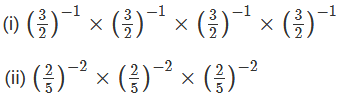Solution:Question: 2

Evaluate: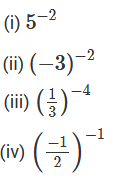Solution: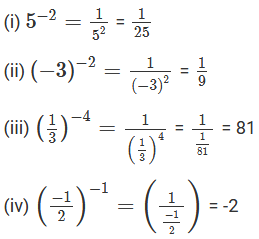Question: 3

Express each of the following as a rational number in the form p/q:Solution: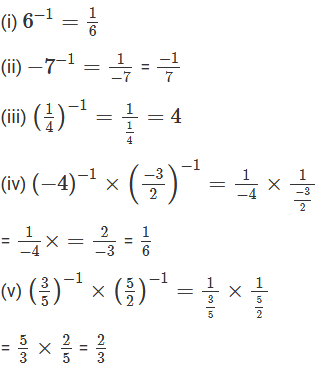Question: 4

Simplify:Solution: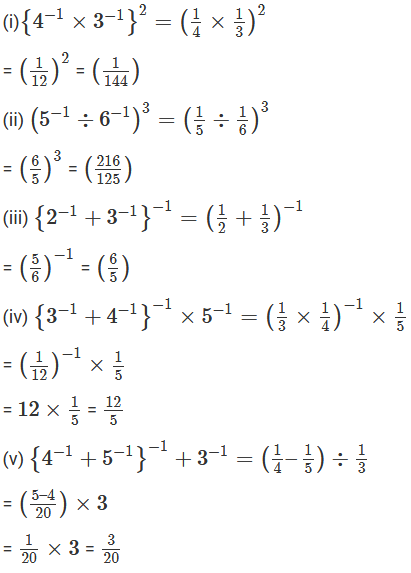Question: 5

Express each of the following rational numbers with a negative exponent: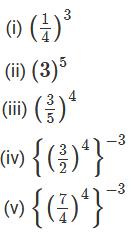Solution: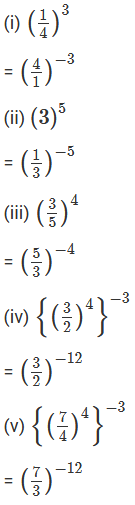Question: 6

Express each of the following rational numbers with a positive exponent.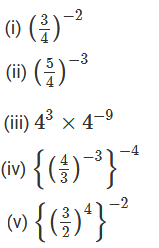Solution: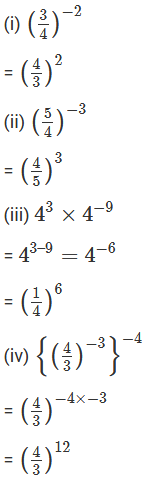Question: 7

Simplify: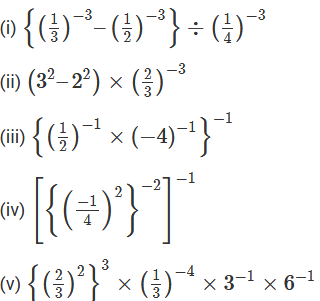Solution: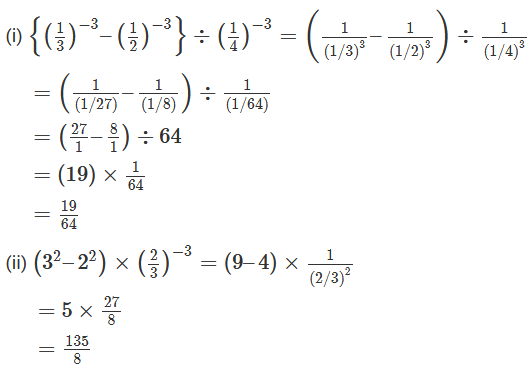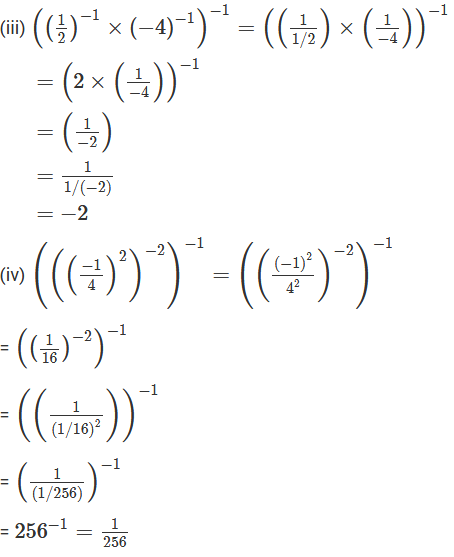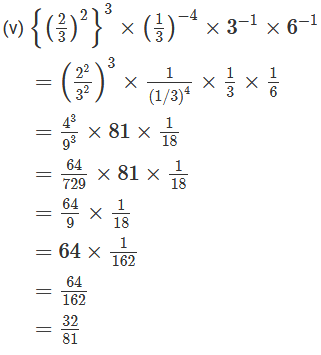Question: 8

By what number should 5-1 be multiplies so that the product may be equal to (–7)-1?

Solution:Question: 9

By what number should (1/2)-1 be multiplies so that the product may be equal to (-4/7)-1?

Solution: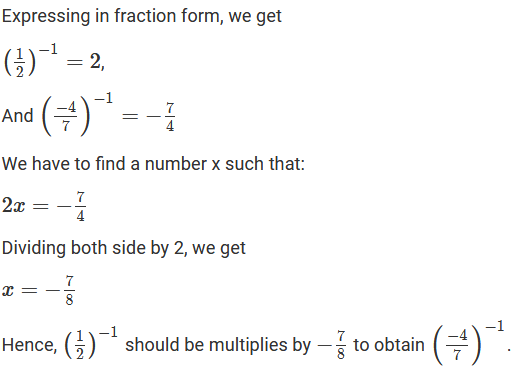Question: 10

By what number should (-15)-1 be divided so that the quotient may be equal to (- 5)-1

Solution: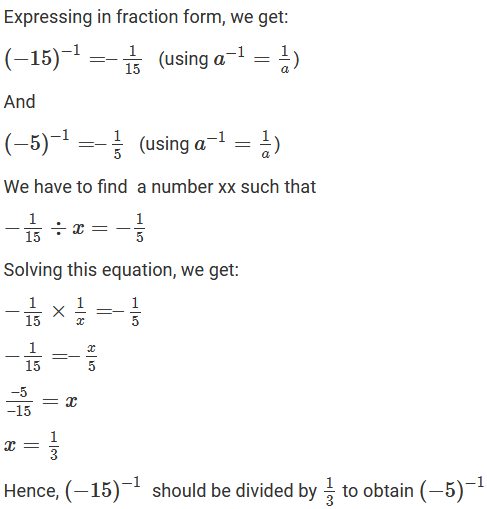Question: 11

By what number should (5/3)-2 be multiplies so that the product may be (7/3)-1?

Solution:Question: 12

Find x, if: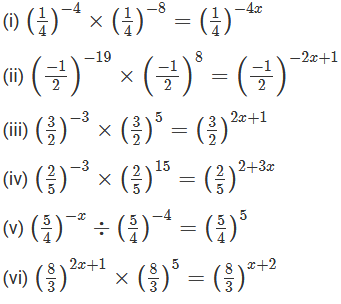Solution: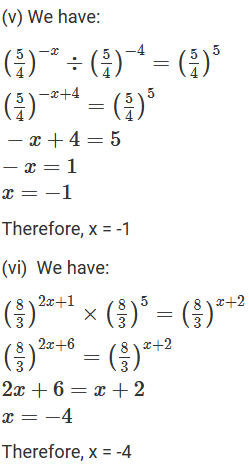Question: 13Solution: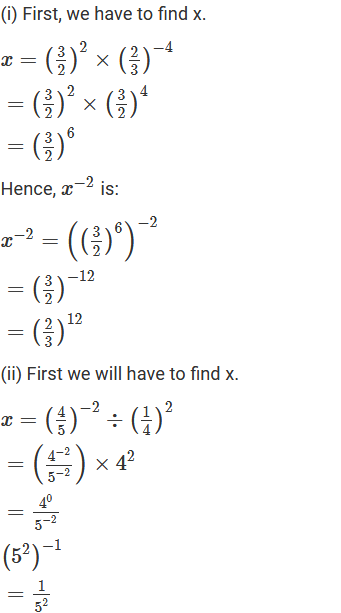Question: 14

Find the value of x for which 52x ÷ 5-3 = 55.

Solution:

52x ÷ 5-3 = 55

52x+3 = 55

2x + 3 = 5

2x = 2

x = 1

Hence, x is 1.### Course Features

• 728 Video Lectures
• Revision Notes
• Previous Year Papers
• Mind Map
• Study Planner
• NCERT Solutions
• Discussion Forum
• Test paper with Video Solution